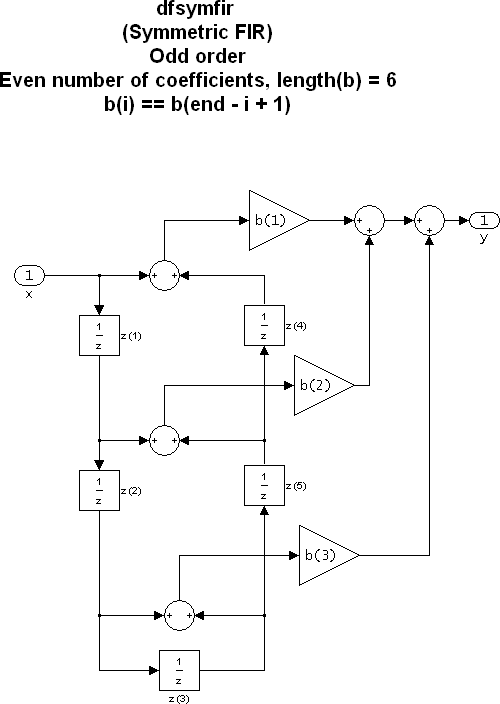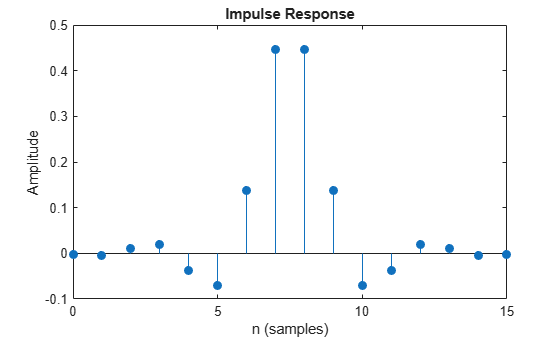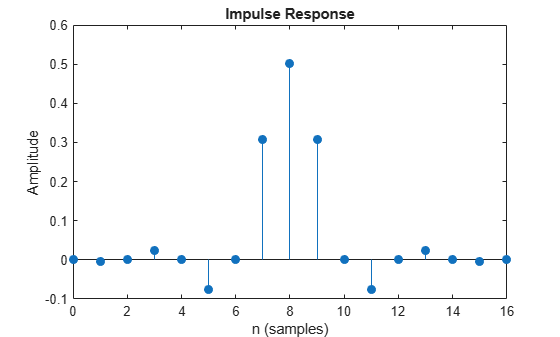# dfilt.dfsymfir

Discrete-time, direct-form symmetric FIR filter

## Syntax

```Hd = dfilt.dfsymfir(b) Hd = dfilt.dfsymfir ```

## Description

`Hd = dfilt.dfsymfir(b)` returns a discrete-time, direct-form symmetric FIR filter, `Hd`, with numerator coefficients `b`.

`Hd = dfilt.dfsymfir` returns a default, discrete-time, direct-form symmetric FIR filter, `Hd`, with `b=1`. This filter passes the input through to the output unchanged.

### Note

Only the first half of vector `b` is used because the second half is assumed to be symmetric. In the figure below for an odd number of coefficients, `b(3)` = 0, `b(4)` = `b(2)` and `b(5)` = `b(1)`, and in the next figure for an even number of coefficients, `b(4)` = `b(3)`, `b(5)` = `b(2)`, and `b(6)` = `b(1)`.The resulting filter states column vector for the odd number of coefficients example above is

`$\left[\begin{array}{c}z\left(1\right)\\ z\left(2\right)\\ z\left(3\right)\\ z\left(4\right)\end{array}\right]$`

## Examples

collapse all

Create a Type-2 15th-order direct-form symmetric FIR filter structure for a `dfilt` object.

```Num_coeffs = fir1(15,0.5); Hd = dfilt.dfsymfir(Num_coeffs);```

Display the impulse response of the filter.

`impz(Hd)`Create a Type-1 16th-order direct-form symmetric FIR filter structure for a `dfilt` object.

```Num_coeffs = fir1(16,0.5); Hd = dfilt.dfsymfir(Num_coeffs);```

Display the impulse response of the filter.

`impz(Hd)`